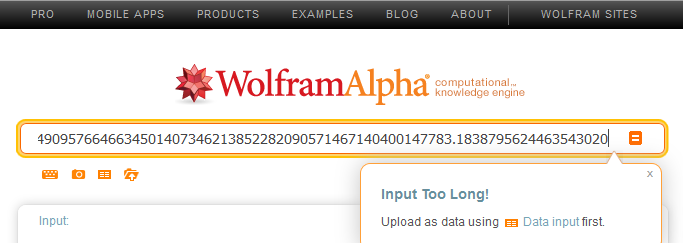Tuesday, March 10, 2015

Quick and Dirty .Net BigDecimal Implementation

[UPDATE 8] Added Conversion, Constructors, Comparisons, Operations for types Short, UShort, Byte, SByte.

[UPDATE 7] Added Ceiling, Floor, Truncate functions as well as fixed the Mod function. Added Ctype to/from byte arrays.
[TODO 3] Implement a BigDecimal rounding function that can round to a specified decimal place.

[UPDATE 6] Added a Random function which returns a random BigDecimal number with the specified number of significant digits.
[TODO 2] Fix modulus of negative numbers.

[UPDATE 5] Fixed constructors for Decimal, Double, and Single as arguments.

[UPDATE 4] Providing the new source code below.

[UPDATE 3] I rewrote the entire thing and improved the speed by ~5x to 10x. Removed all string manipulation hacks for calculations and added several other things for ease of use in code. I compiled it to a dll for ease of use.
Usage:
Reference the BigDecimal.Dll,
"Import TizzyT"
Optionally you can also "Import TizzyT.BigDecimal"
Ex:
Dim bigNumber as BigDecimal = "51316561513854163416165416163163165613.1473415424496255785965457458965348754854856"
or
Dim bigNumber as New BigDecimal("51316561513854163416165416163163165613.1473415424496255785965457458965348754854856")
The constructors accepts these types as the parameter:
String, BigInteger, Decimal, Double, Integer, Long, Single, UInteger, ULong.
Supported Operators: /, *, -, +, ^, Mod, =, <, >, <=, >=, <>
There are no BitWise operators.

[TODO 1] Add e constant calculation function and Phi calculation function. Add pre-calculated values to the first 65535 digits for Pi and e (and other constants in the future) for fast lookup and only calculating when needed. Fix potential rounding issue in the divide function. Support parsing and simple calculations with imaginary numbers. Raising to decimal powers.

[UPDATE 2] Added Pi function which (calculates Pi to the specified decimal place).

[UPDATE 1] Added square root function (calculates the square root to a specified decimal place).

Now many of us I'm sure have gone to Wolfram Alpha and have done some calculations and get very precise answers. I thought why cant I just make a calculator that runs on the computer instead of relying on a website. The problem here is that I typically write in .Net and as far as I know in .Net there isn't yet a BigDecimal implementation though I hear something called Rational Number will be in the future (correct me if I'm wrong). .Net as of 4.5 does include BigInteger though and I figured "hey I can surely manipulate that to do decimals". So by playing with powers of 10 and some string manipulation I threw together this quick and dirty BigDecimal implementation. It does most of the basic arithmetic operations (add, subtract, multiply, divide) and also includes modulus and a limited power operator (only decimals to integer powers allowed).
It also has most of the basic comparison operators (=,<>,>,<,>=,<=).

I didn't implement a way to do powers (exponents) of decimal numbers to decimal numbers as I don't know of any way to do arithmetic that are to the power of a decimal number. This is one of many things Wolfram Alpha and other tools are still useful for. I did however add a power operator where you can raise a decimal number to an integer power. Again this was a quick and dirty implementation by use of an already existing BigInteger and some string manipulation so this is more of a workaround then a full and proper implementation. With all that said this works as it is intended and I just thought I'd share this for those who want to have such capabilities and/or not want to make their own or use one that others have made (for what ever reason). Below is the source in VB.net, feel free to critique it constructively or even suggest improvements/additions/corrections/other changes (they are always welcome) in the comments, even link to other/better implementations for others to learn from (including myself lol).

Here is a brief and simplified explanation of how the operators work (take note of the number of digits after the decimal places, trailing zeros after the decimal are omitted).
ex: 2.5 + 3.56
250 + 356 = 606
606 * 10^-2 = 6.06
How Subtraction is done:
ex: 3.56 - 2.5
356 - 250 = 106
106 * 10^-2 = 1.06
How Mulitplication is done:
ex: 1.23 * 2.34
123 * 234 = 28782
28782 * 10^-4 = 2.8782
How Division is done:
ex: 2.75 / 1.23
27500 / 123 = 223.5772...
223.5772... * 10^-2 = 2.235772...
How Modulus is done:
ex: 81.913 mod 1.89
81913 mod 1890 = 643
643 * 10^-3 = 0.643
How Powers is done:
ex: 3.86 ^ 3
386 ^ 3 = 57512456
57512456 * 10^(-2 * 3) = 57.512456

Here is an example of what this BigDecimal can do:
-29904881154799326178521321561318483083459729644316160000768321084631624262879277725524837232512874501269189723158150646979348274909576646634501407346213852282090571467140400147783_._18387956244635430200491958026044099076018957040468456891742982019954636328243381756769819732303584718583212854736122624014489392565966217355695509628096970419381029887990526931898967457998680807367672273756904951032467189830062966412679369009462886580958333279494754935309708946817547646334519060461784118024038735185710860189063748402342861130577
*
5751924938051280223833858629501675273310948056012555014190229443738691691398479261795665007308040432731195362671005864806268338234894643060929333520217662547275713887745280398692580701613219578511545688593727384579400216343571287604699210898682409901033923592254977998928042776007128567167661764461451197309014749502640082135628831465593026815282225172036806167117327391851426784340266832879686969785482431161011282785148552226455709885783477185740418454692010461531139699177137747603724519366291555351081827755537285870661022388558239662190786473991577468995843919739761368997465938707076067624686764323911947153991511165379474331079151578602891362316550805454661607383094255347700102452925611494129497831220455765356914545838285121830226022147084604811_._485209203433392412988139774358020034502772971469229293225542494540235780020626731513983670906928733123343

Wolfram Alpha would show:This will give:
-172010631683750011631176079635100632512013244572007775432003052061741528932456706083001536611193096484622166575907367022582529375403056568752074822302755095141705749035603368873993087184637206623936125077741562315269979582630307542103138837485919587574525593555179880851697392546867297871962651246670873381798031600455987658621905814271481025010371299023464068557582075120843141692643569603040719610403883441172974614752898206095324363859673141540896718756076235625588707331308369632854757249392548068974677743079393957197900647731970295656741222088267367125066341257683453363029468157717943954078353804877795513312209888179482890880751242235524454941490185521697979596299416656404943757921088267838665435536479783956085537537344245358014725997793150006008217624566831017875248663228026355877838648274813511813170883984822985170888041944419334079113344858945397015576199007001645366831336899975261719765525043062998876285207094159601_._64451178007533823634375369013823879310973576406237333385810760066596575526779234269950974308196349757681037340570364428921472113903604913391171361506598161298515062927800899848793991527144615663644225257812312313442943022512764968630618332043281176249948189766049626681715362863353932346845491296791798272977703881944317699353132567214725666489749891407117892159573738750826924897100577519286872165820424322663912528782448439048590873529321689369758911

New Source:

Old Source: http://pastebin.com/embed_js.php?i=jcNaUhuH

Free for non commercial use. All changes to source must be provide here.